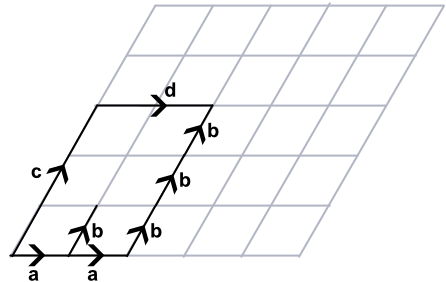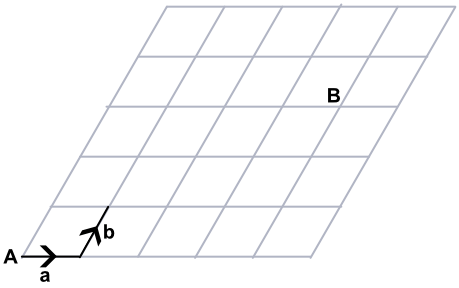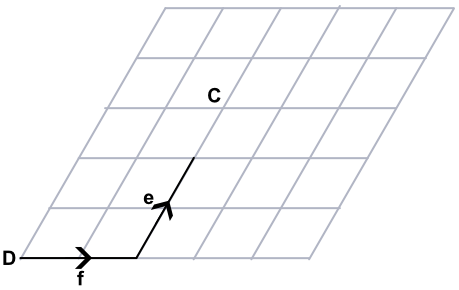Multiplication of Vectors

## Multiplication of Vectors

Vectors can be multiplied.

a + a + a = 3a

The multiplier of the vector is called a scalar. A scalar can take any numeric value, including negative amd fractional values.In the example above, c = 3b. Similarly, a = frac(1)(2)d.

## Example 1

State the resultant vector vec(AB) in terms of a and b.Four instances of a along; and three instances of b up.

Answer: 4bb(a) + 3bb(b)

## Example 2

State the resultant vector vec(CD) in terms of e and f.To get from C, move 1frac(1)(2) worth of e in the opposite direction; and 1 worth of f in the opposite direction.